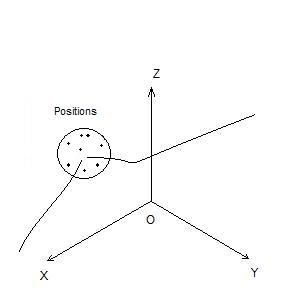# Vertex defined with multiple positions

## Detailed Description

group a3d_multiple_vertex

Entity type is kA3DTypeTopoMultipleVertex.

A multiple vertex is defined as the average position of several 3D points, which are typically the endpoints of edge curves that end at this vertex.Warning

In general, no constraint is given to the 3D points stored in the array referenced by the A3DTopoMultipleVertexData::m_pPts member. In particular, the same point (same coordinates) may appear multiple times in that array. This is a common behavior, and all these points must be taken into account to evaluate the tolerance.

## Function Documentation

A3DStatus A3DTopoMultipleVertexGet(const A3DTopoMultipleVertex *pMultipleVertex, A3DTopoMultipleVertexData *pData)

Populates the A3DTopoMultipleVertexData structure.

Version

2.0

Return values
• A3D_INITIALIZE_NOT_CALLED

• A3D_INVALID_DATA_STRUCT_SIZE

• A3D_INVALID_DATA_STRUCT_NULL

• A3D_INVALID_ENTITY_NULL

• A3D_INVALID_ENTITY_TYPE

• A3D_SUCCESS

Returns

A3D_SUCCESS in case of success or an error code

A3DStatus A3DTopoMultipleVertexCreate(const A3DTopoMultipleVertexData *pData, A3DTopoMultipleVertex **ppMultipleVertex)

Creates an A3DTopoMultipleVertex from an A3DTopoMultipleVertexData structure.

Version

2.0

Return values
• A3D_INVALID_DATA_STRUCT_SIZE

• A3D_INVALID_DATA_STRUCT_NULL

• A3D_SUCCESS

Returns

A3D_SUCCESS in case of success or an error code